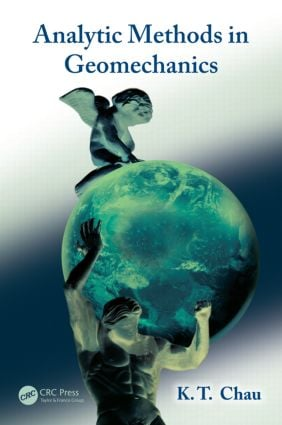# Analytic Methods in Geomechanics

## 1st Edition

CRC Press

457 pages | 177 B/W Illus.

Paperback: 9781466555853
pub: 2012-12-06
SAVE ~\$16.99
\$84.95
\$67.96
x
eBook (VitalSource) : 9781315275277
pub: 2018-10-08
from \$39.48

FREE Standard Shipping!

### Description

A multidisciplinary field, encompassing both geophysics and civil engineering, geomechanics deals with the deformation and failure process in geomaterials such as soil and rock. Although powerful numerical tools have been developed, analytical solutions still play an important role in solving practical problems in this area. Analytic Methods in Geomechanicsprovides a much-needed text on mathematical theory in geomechanics, beneficial for readers of varied backgrounds entering this field.

Written for scientists and engineers who have had some exposure to engineering mathematics and strength of materials, the text covers major topics in tensor analysis, 2-D elasticity, and 3-D elasticity, plasticity, fracture mechanics, and viscoelasticity. It also discusses the use of displacement functions in poroelasticity, the basics of wave propagations, and dynamics that are relevant to the modeling of geomaterials. The book presents both the fundamentals and more advanced content for understanding the latest research results and applying them to practical problems in geomechanics.

The author gives concise explanations of each subject area, using a step-by-step process with many worked examples. He strikes a balance between breadth of material and depth of details, and includes recommended reading in each chapter for readers who would like additional technical information. This text is suitable for students at both undergraduate and graduate levels, as well as for professionals and researchers.

Elementary Tensor Analysis

Introduction

General Tensors, Cartesian Tensors, and Tensor Rank

A Brief Review of Vector Analysis

Dyadic Form of Second Order Tensors

Derivatives of Tensors

Divergence and Stokes Theorems

Some Formulae in Cylindrical Coordinates

Some Formulae in Spherical Coordinates

Problems

Elasticity and Its Applications

Introduction

Basic Concepts for Stress Tensor

Piola–Kirchhoff Stresses

Coordinate Transformation of Stress

Basic Concepts for Strain Tensor

Rate of Deformation

Compatibility Equations

Hill’s Work-conjugate Stress Measures

Constitutive Relation

Isotropic Solids

Transversely Isotropic Solids

Equations of Motion and Equilibrium

Compatibility Equation in Terms of Stress Tensor

Strain Energy Density

Complementary Energy

Hyperelasticity and Hypoelasticity

Plane Stress, Plane Strain and the Airy Stress Function

Stress Concentration at a Circular Hole

Force Acting at the Apex of a Wedge

Solution for Indirect Tensile Test (Brazilian Test)

Jaeger’s Modified Brazilian Test

Edge Dislocation

Dislocation Pile-up and Crack

Screw Dislocation and Faulting

Mura Formula for Curved Dislocation

Problems

Complex Variable Methods for 2-D Elasticity

Introduction

Coordinate Transformation in Complex Variable Theory

Homogeneous Stresses in Terms Analytic Functions

A Borehole Subject to Internal Pressure

Kirsch Solution by Complex Variable Method

Definiteness and Uniqueness of the Analytic Function

Boundary Conditions for the Analytic Functions

Single-valued Condition for Multi-connected Bodies

Multi-connected Body of Infinite Extend

General Transformation of Quantities

Elastic Body with Holes

Stress Concentration at a Square Hole

Mapping Functions for Other Holes

Problems

Three-Dimensional Solutions in Elasticity

Introduction

Displacement Formulation

Stress Formulations

Some 3-D Solutions in Geomechanics

Harmonic Functions and Indirect Method

Harmonic Functions in Spherical Coordinates

Harmonic Functions in Cylindrical Coordinates

Biharmonic Functions

Muki’s Formulation in Cylindrical Coordinates

Problems

Plasticity and Its Applications

Introduction

Flow Theory and Deformation Theory

Yield Function and Plastic Potential

Elasto-plastic Constitutive Model

Rudnicki–Rice (1975) Model

Drucker’s Postulate, PMPR, and Il’iushin’s Postulate

Yield Vertex

Mohr–Coulomb Model

Lode Angle or Parameter

Yield Criteria on the π-Plane

Other Soil Yield Models

Cap Models

Physical Meaning of Cam-Clay Model

Modified Cam-Clay

A Cam-Clay Model for Finite Strain

Plasticity by Internal Variables

Viscoplasticity

Problems

Fracture Mechanics and Its Applications

Introduction

Stress Concentration at a Elliptical Hole

Stress Concentration at a Tensile Crack

Stress Field near a Shear Crack

The General Stress and Displacement Field for Mode I Cracks

The General Stress and Displacement Field for Mode II Cracks

The General Stress and Displacement Field for Mode III Cracks

The Energy Release Rate at Crack Tips

Fracture Toughness for Rocks

J-integral and the Energy Release Rate

Westergaard Stress Function and Superposition

Growth of Slip Surface in Slopes

Energy Release Rate for Earthquake

Wing Crack Model under Compressions

Bazant’s Size Effect Law via J-integral

Continuum Damage Mechanics

Solids Containing Microcracks

Rudnicki–Chau (1996) Multiaxial Microcrack Model

Problems

Viscoelasticty and Its Applications

Introduction

Boltzmann’s Integral Form of Stress and Strain

Stieltjes Convolution Notation

Stress-Strain Relation in Differential Equation Form

Stress-strain Relation in Laplace Transform Space

Correspondence Principle

Creeping and Relaxation Tests

Calibration of the Viscoelastic Model

Viscoelastic Crack Models for Steam Injection

Problems

Linear Elastic Fluid-Infiltrated Solids and Poroelasticity

Introduction

Biot’s Theory of Poroelasticity

Biot–Verruijt Displacement Function

McNamee–Gibson–Verruijt Displacement Function

Schiffman–Fungaroli–Verruijt Displacement Function

Schiffman–Fungaroli Displacement Function

Laplace–Hankel Transform Technique

Point Forces and Point Fluid Source in Half-space

Cleary’s Fundamental Solution of Point Forces in Full Space

Rudnicki’s Fundamental Solutions in Full Space

Thermoelasticity vs. Poroelasticity

Problems

Dynamics and Waves In Geomaterials

Introduction

Seismic Waves

Waves in Infinite Elastic Isotropic Solids

Helmholtz Theorem and Wave Speeds

Rayleigh Waves

Love Waves

Stoneley Waves

Elastic-plastic Waves

Waves in Viscoelastic Solids

Dynamic Fracture Mechanics

Vibrations and Soil Dynamics

Problems

Appendices

Appendix A: Nanson Formula

Appendix B: Laplace Transform

Appendix C: Legendre Transform and Work Increments

Selected Biographies

References

Author Index

Subject Index

#### Chau K.T.

Kowloon, HKSAR, China